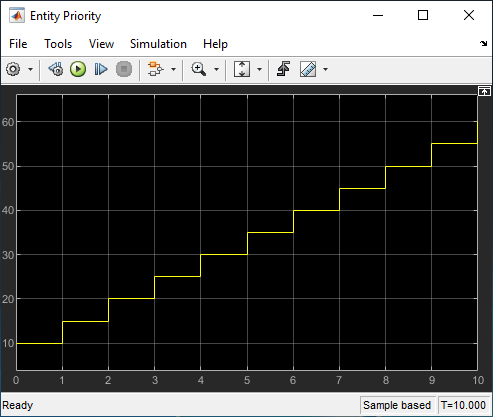## Custom Entity Generator Block with Signal Input and Signal Output

This example shows how to create a custom source block that generates entities and to manage discrete states when implementing the discrete-event System object™ methods.

Suppose that you manage a facility that produces raw materials with a fixed deterministic rate. The materials contain a 12-digit bar code for stock management and priority values for order prioritization. To represent this behavior, this example shows how to generate a custom entity storage block is generated with one signal input port, one entity output port, one signal output port, and one storage element. The block generates entities with distinct priority values. The entities carry data and depart the block from its output port. The entity priority values are acquired from values of the incoming signal.

To open the model and to observe the behavior of the custom block, see `CustomEntityGeneratorBlockExample`.

### Create the Discrete-Event System Object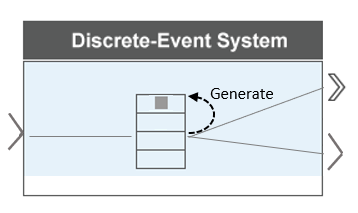The block is defined as a custom entity generator block that generates entities with specified intergeneration periods. The generated entities carry data, and their priority values are determined by the values of the input signal.

### Custom Block Behavior

1. Define the time between material generations.

``` % Nontunable properties properties (Nontunable) % Generation period period = 1; end```
2. Initialize the discrete state variables.

``` function resetImpl(obj) % Initialize / reset discrete-state properties. obj.priority = 10; obj.value = 1:12; end ```

The variable `priority` represents material priority and the `value` represents bar code data carried by the materials.

3. Initialize the output for a source block.

``` function num = getNumOutputsImpl(~) % Define total number of outputs. num = 2; end function [out1 out2] = getOutputSizeImpl(obj) % Return size for each output port. out1 = [1 12]; out2 = 1; end function [out1 out2] = getOutputDataTypeImpl(obj) % Return data type for each output port. out1 = "double"; out2 = "double"; end function [out1 out2] = isOutputComplexImpl(obj) % Return true for each output port with complex data. out1 = false; out2 = false; end ```
• First function declares the output size.

• Second function declares that output port data types are `double`.

• Third function declares `false` for output ports because they do not support complex data.

4. Declare the size, data, and complexity of the discrete states.

``` function [sz,dt,cp] = getDiscreteStateSpecificationImpl(obj,name) % Return size, data type, and complexity of discrete-state. switch name case 'priority' sz = [1 1]; case 'value' sz = [1 12]; end dt = "double"; cp = false; end```
• The discrete state `priority` is scalar. The data type is `double` and takes real values.

• The discrete state `value` is a `1`-by-`12` vector. The data type is `double` and takes real values.

5. Generate the materials with intergeneration period, priority, and data defined by:

• The parameter `obj.period`, declared as a public parameter that can be changed from the block dialog box.

• The parameter `obj.priority` values, defined by the signal from the input port.

• The parameter `obj.value`, a `1`-by-`12` vector which represents the data carried by entities.

``` function events = setupEvents(obj) % Set up entity generation event for storage 1 at simulation start. events = obj.eventGenerate(1,'mygen',obj.period,obj.priority); % Set up the initial value of the output signal. out1 = 10; end function [entity,events,out1] = generate(obj,storage,entity,tag,in1) % Specify event actions when entity is generated in storage. entity.data = obj.value; % The value from the signal is assigned to the entity priority. obj.priority = in1; % Output signal is the assigned priority value. out1 = obj.priority; events = [obj.eventForward('output',1,0) ... obj.eventGenerate(1,'mygen',obj.period,obj.priority)]; end```

### Implement Custom Block

1. Save the `.m` file as `CustomEntityStorageBlockGeneration`. Link the System object to a SimEvents® model by using a MATLAB Discrete-Event System block. For more information about linking, see Create Custom Blocks Using MATLAB Discrete-Event System Block.

2. Create a SimEvents model that includes the MATLAB Discrete-Event System block, a Ramp block, an Entity Terminator block, and two Scope blocks. Connect the blocks as shown in the model.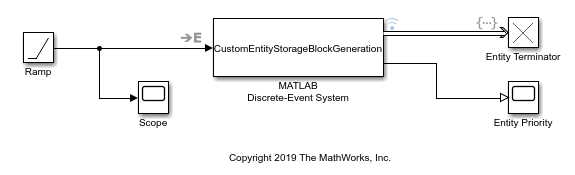3. In the Ramp block, set Slope to `5` and Initial output to `10`.

4. In the Entity Terminator block, you can display the priority values of the entities arriving at the block, in the Entry action field enter this code.

```coder.extrinsic('fprintf'); fprintf('Priority: %d\n', double(entitySys.priority))```
5. Right-click the entity path from the custom Entity Generator to the Entity Terminator and select the .

6. Simulate the model.

1. Observe the output of the Ramp block. For instance, the output value becomes `15`, `20`, `25`, and `30` for the simulation time `1`, `2`, `3`, and `4`, respectively.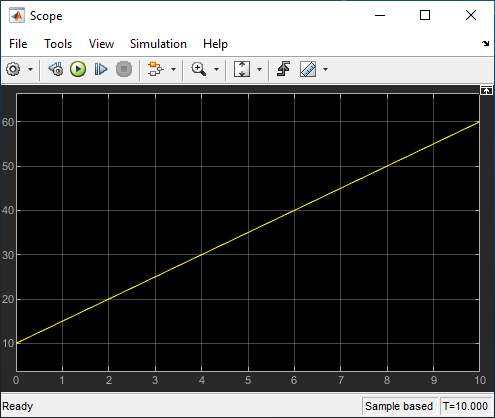2. The Simulation Data Inspector shows that entities are forwarded to the Entity Terminator block with data of size 1-by-12.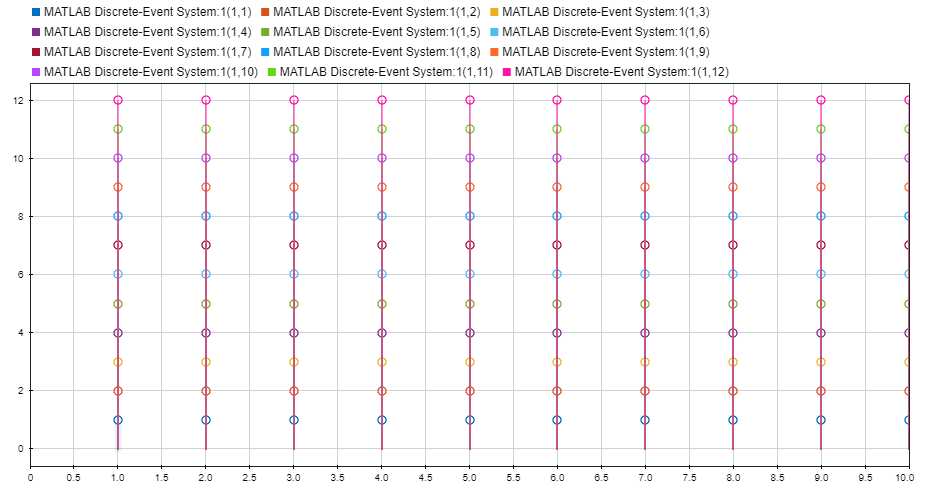3. You can also observe the priority values from the scope labeled Entity Priority for generation times `1`, `2`,`3`, `4`, `5`, `6`, `7`, `8`, `9`, and `10`.